Vibroengineering PROCEDIA

Published: 19 October 2020

# An improved mesh stiffness calculation model of spur gear pair under mixed EHL friction with spalling effect

Siyu Wang1
Rupeng Zhu2
1, 2College of Mechanical and Electrical Engineering, Nanjing University of Aeronautics and Astronautics, Nanjing, 210016, China
1, 2National Key Laboratory of Science and Technology on Helicopter Transmission, Nanjing University of Aeronautics and Astronautics, Nanjing, 210016, China
Corresponding Author:
Rupeng Zhu
Views 247

#### Abstract

Based on the “Potential energy method”, the improved mesh stiffness calculation model of spur gear pair with actual involute and transition curve is proposed. Furthermore, the spalling effect, nonlinear contact stiffness, improved fillet-foundation stiffness, time-varying friction coefficient under mixed elasto-hydrodynamic lubrication (EHL) condition are assimilated into the model. Then the results of the improved mesh stiffness model are obtained by numerical simulation, in which the mesh stiffness under mixed EHL friction and the effect of height for spalled area on the mesh stiffness are comprehensively investigated.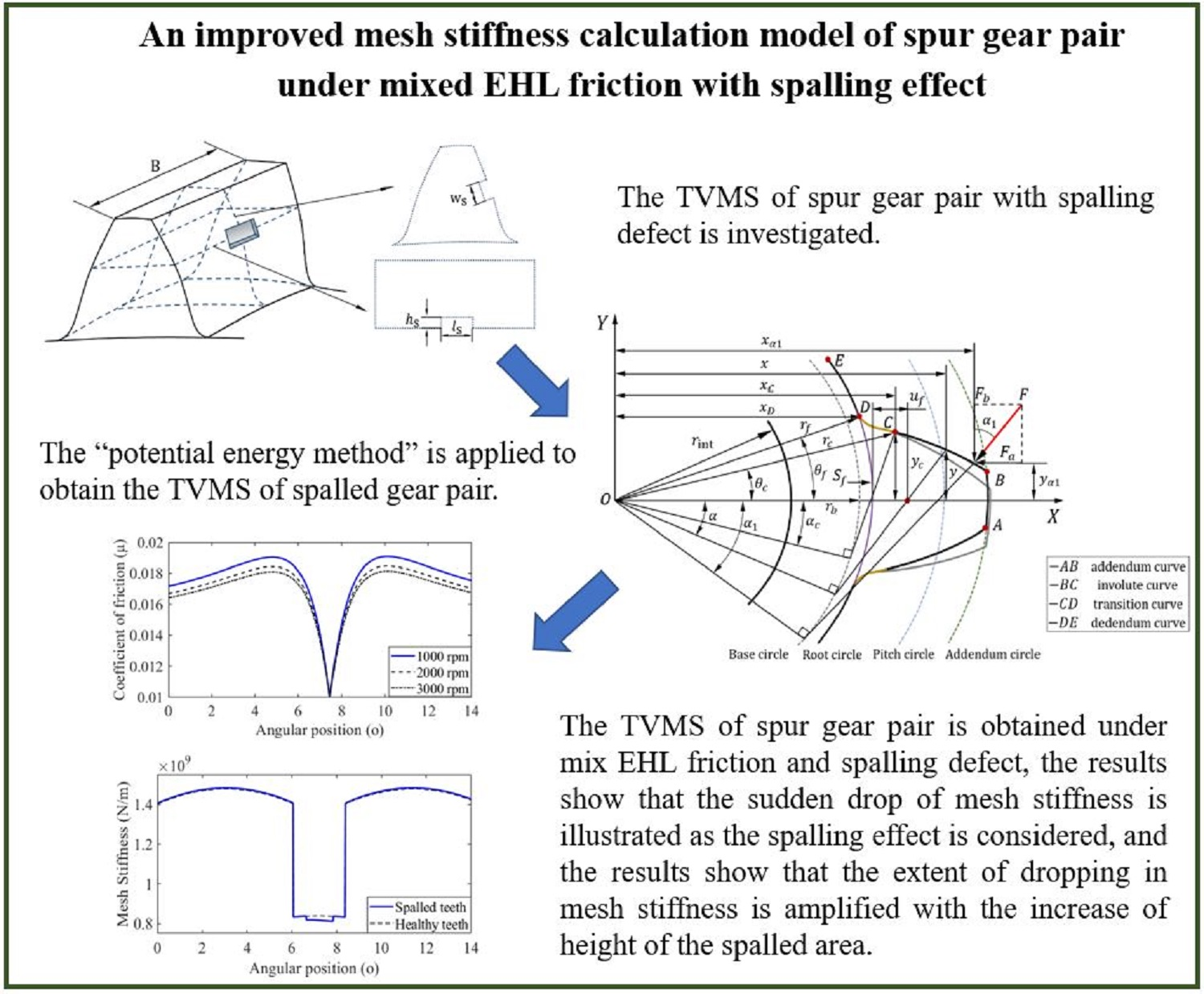#### Highlights

• The improved model of TVMS calculation for spur gear pair with spalling defect is proposed.
• The time-varying friction force under mixed EHL condition is considered in TVMS calculation.
• The influence of multiple spalling parameters on the TVMS of spur gear pair is studied.

## 1. Introduction

Time-varying meshing stiffness (TVMS) is an important stimulating factor of the gear system, the vibration and noise of the gear system are greatly affected by the meshing stiffness. Therefore, in order to obtain the TVMS in accurate and efficient way, many improved and modified methods have been proposed. The analytical method to calculate the gear tooth deflections was proposed in . The nonlinear Hertzian contact stiffness was proposed based on . Yang et al.  studied gear impact dynamics with Hertzian damping, tooth friction, and bending stiffness. Zhu et al.  designed a program to predict the lubrication features, friction, surface stress and flash temperature based on measured 3D surface roughness. Castro et al.  obtained the coefficient of friction in mixed film lubrication based on the model of gears with discs. Chaari et al.  studied the mesh stiffness and dynamic response of a spur gear system under the effect of spalling and tooth breakage. Chen et al.  conducted dynamic simulation of spur gear, in which the tooth root crack propagates along tooth width and crack depth. Kiekbusch et al.  calculated the torsional mesh stiffness of spur gears based on 2D and 3D FE models. Chen et al.  conducted dynamic analysis for the spur gear with crack in the gear tooth, and the crack depth on the system response was also analyzed. Wan et al.  improved the calculation process of mesh stiffness, and the dynamic model of gear-rotor system was proposed considering tooth root crack. Ma et al.  proposed a dynamic model for cracked spur gear, then the time-varying stiffness was calculated based on the model. Wang et al.  built a model of spur gear pair with profile error, and then the mesh characteristics were studied. Liang et al.  evaluated the mesh stiffness of planetary gear set under the influence of crack. Saxena et al. [14,15] studied the influence of shaft misalignment and friction on the mesh stiffness of spur gear pair. Ma et al. proposed improved time-varying mesh stiffness model of spur gears under cracked effect , and with tip relief .

In the paper, the mesh stiffness calculation model of spur gear pair with spalling effect is proposed, in which the exact involute and transition curves of gear teeth, the nonlinear Hertzian contact stiffness, modified fillet-foundation stiffness, mixed EHL friction coefficient are fully considered.

## 2. Analytical method of TVMS for spur gear pair

The tooth profile of the spur gear enters and leaves contact simultaneously along the entire width of the end face. Therefore, when the tooth profile comes into and out of contact, this will cause sudden loading and unloading on the teeth. As a result, vibration and noise are generated.

The “potential energy method” [3,9] is applied in the study to obtain the TVMS of spur gear pair under mixed EHL friction excitation with spalling effects. The gear deformation consists of three parts of elastic deformation: 1. the bending deformation, shear deformation, and axial compression deformation of the tooth portion; 2. the base deformation of the transition fillet connected to the gear teeth; 3. the contact deformation between the tooth causing the contact stress. Therefore, the corresponding mesh stiffness components can be derived from the gear deformations.

### 2.1. Friction coefficients obtained by mixed elasto-hydrodynamic lubrication

Under mixed EHL condition, the synthesized coefficient of friction ${\mu }_{ML}$ can be obtained throught following expression :

1
${\mu }_{ML}=\left(1-{f}_{a}\right){\mu }_{BL}+{f}_{a}{\mu }_{EL},$

where, ${\mu }_{BL}$, ${\mu }_{EL}$ indicate the coefficient of friction in the boundary film lubrication and full film lubrication, respectively. ${f}_{a}$ represents the load distribution function, which determined by the specific lubricating film thickness between gear teeth. For the boundary film lubrication condition, the coefficient of friction is in the interval 0.08-0.13, and ${\mu }_{BL}$ = 0.1 is adopted in the study.

For the full film lubrication condition , the coefficient of fiction can be calculated by:

2
${\mu }_{EL}={e}^{f\left(SR,{P}_{h},{v}_{0},S\right)}{P}_{h}^{{b}_{2}}{\left|SR\right|}^{{b}_{3}}{V}_{e}^{{b}_{6}}{v}_{0}^{{b}_{7}}{R}^{{b}_{8}},$
3
$f\left(SR,{P}_{h},{v}_{0},S\right)={b}_{1}+{b}_{4}\left|SR\right|{P}_{h}\mathrm{l}\mathrm{o}{\mathrm{g}}_{10}\left({v}_{0}\right)+{b}_{5}{e}^{-\left|SR\right|{P}_{h}\mathrm{l}\mathrm{o}{\mathrm{g}}_{10}\left({v}_{0}\right)}+{b}_{9}{e}^{S},$

where, ${b}_{i}$ are constant coefficients that shown in Table 2, $SR$ indicates the slide-roll ratio, indicated the maximum Hertzian pressure, ${v}_{0}$ presents the absolute viscosity, and $R$ denotes the effective curvature radius, ${v}_{e}$ denotes the suction speed at the contact point.

### 2.2. Analytical method of gear tooth stiffness

The Fig. 1 shows the schematic diagram of spur gear tooth under meshing force, Fig. 2 shows the spur gear tooth with spalling effect. With angular displacement adopted, the tooth axial compressive stiffness, tooth bending stiffness, and tooth shear stiffness and can be expressed as:

4
$\frac{1}{d{k}_{t\mathrm{a}}}={\int }_{\pi /2}^{{\alpha }_{t}}\frac{{\left(\mathrm{s}\mathrm{i}\mathrm{n}{\alpha }_{1}±\mu \mathrm{c}\mathrm{o}\mathrm{s}{\alpha }_{1}\right)}^{2}}{E{A}_{x1}}\frac{d{x}_{1}}{d\gamma }d\gamma +{\int }_{{\alpha }_{c}}^{{\alpha }_{1}}\frac{{\left(\mathrm{s}\mathrm{i}\mathrm{n}{\alpha }_{1}±\mu \mathrm{c}\mathrm{o}\mathrm{s}{\alpha }_{1}\right)}^{2}}{E{A}_{x2}}\frac{d{x}_{2}}{d\tau }d\tau ,$
5
$\frac{1}{d{k}_{tb}}={\int }_{\pi /2}^{{\alpha }_{t}}\frac{{\left[\left(\mathrm{c}\mathrm{o}\mathrm{s}{\alpha }_{1}\mp \mu \mathrm{s}\mathrm{i}\mathrm{n}{\alpha }_{1}\right)\left({x}_{\alpha 1}-{x}_{1}\right)-{y}_{\alpha 1}\left(\mathrm{s}\mathrm{i}\mathrm{n}{\alpha }_{1}±\mu \mathrm{c}\mathrm{o}\mathrm{s}{\alpha }_{1}\right)\right]}^{2}}{E{I}_{x1}}\frac{d{x}_{1}}{d\gamma }d\gamma$
6
$\frac{1}{d{k}_{ts}}={\int }_{\pi /2}^{{\alpha }_{t}}\frac{1.2{\left(\mathrm{c}\mathrm{o}\mathrm{s}{\alpha }_{1}\mp \mu \mathrm{s}\mathrm{i}\mathrm{n}{\alpha }_{1}\right)}^{2}}{G{A}_{x1}}\frac{d{x}_{1}}{d\gamma }d\gamma +{\int }_{{\alpha }_{c}}^{{\alpha }_{1}}\frac{1.2{\left(\mathrm{c}\mathrm{o}\mathrm{s}{\alpha }_{1}\mp \mu \mathrm{s}\mathrm{i}\mathrm{n}{\alpha }_{1}\right)}^{2}}{G{A}_{x2}}\frac{d{x}_{2}}{d\tau }d\tau ,$

where, ${x}_{\alpha 1}$, ${y}_{\alpha 1}$, ${x}_{1}$, ${y}_{1}$, ${x}_{2}$, ${y}_{2}$ can be described in function of angles $\alpha$, $\gamma$, $\tau$ . In the of double operators $±$ and $\mp$ in above expressions, the upper operators represent the approach process, the lower operators represent recess process. The cross-sectional area of spalling area can be expressed as ${A}_{s}=\left(2\cdot {h}_{x}\cdot L\right)-\left({h}_{s}\cdot {l}_{s}\right)$, and the corresponding moment of inertia of the section can be calculated by ${I}_{s}=1/12\cdot \left[{\left(2\cdot {h}_{x}\right)}^{3}\cdot L-\left({h}_{s}^{3}\cdot {l}_{s}\right)\right]$.

Fig. 1Schematic diagram of spur gear tooth under meshing force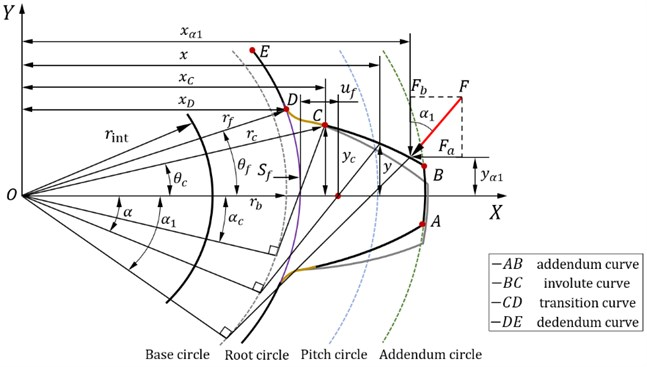Fig. 2Schematic diagram of spur gear tooth with spalling effect and spalling parameters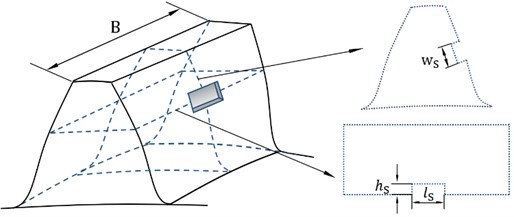### 2.3. Analytical method of nonlinear contact stiffness

Due to the nonlinear contact force between gear tooth, the Hertzian contact stiffness is nonlinear in principle. The analytical method is applied to express the nonlinear Hertizian contact , therefore, the approximate formula is drawn as follows:

7
${k}_{hi}=\frac{{E}_{e}^{0.9}{L}^{0.8}{\left({F}_{i}\right)}^{0.1}}{1.275},$
8
${F}_{i}=F\cdot \left(Ls{r}_{i}\right),$

where, $i$ denotes the $i$th meshing tooth pair, $L$ is the tooth face width, $F$ is the total meshing force, which can be expressed as $T/{r}_{b1}$, where, $T$ denotes the torque of the driving gear, ${F}_{i}$ is the meshing force of the $i$th tooth pair.

### 2.4. Analytical method of improved gear foundation stiffness

When calculating the mesh stiffness, in addition to tooth deformation, the deflection caused by the fillet foundation should also be considered. The stiffness component caused by the deflection of the fillet foundation can be expressed as:

9
$\frac{1}{d{k}_{f}}=\frac{\mathrm{c}\mathrm{o}{\mathrm{s}}^{2}{\alpha }_{1}}{E\cdot dL}\left\{{L}^{\mathrm{*}}{\left(\frac{{u}_{f}}{{S}_{f}}\right)}^{2}+{M}^{\mathrm{*}}\left(\frac{{u}_{f}}{{S}_{f}}\right)+{P}^{\mathrm{*}}\left(1+{Q}^{\mathrm{*}}\mathrm{t}\mathrm{a}{\mathrm{n}}^{2}{\alpha }_{1}\right)\right\},$

where, $L$ is the tooth width of spur gear, ${\alpha }_{1}$ is the pressure angle acting on gear tooth, ${u}_{f}$ and ${S}_{f}$ are illustrated in schematic diagram, the coefficients ${L}^{*}$, ${M}^{*},$${P}^{*}$, ${Q}^{*}$ can be obtained from the polynomial functions proposed in .

In the actual condition, one gear body support multiple gear tooth in meshing, therefore, higher value of mesh stiffness is obtained, that compared with the actual value in multiple-tooth meshing.

An improved analytical method based on FEM simulation is proposed  to eliminate the deviation, which can be expressed as:

10

where, $\lambda$ denotes the correction coefficient for fillet-foundation stiffness, $p$ and $g$ indicate the driving, driven gear, respectively, and $N$ presents the number of gear pairs in meshing.

## 3. Results and discussion

The basic parameters of the spur gear pair that used for improved TVMS calculation model is shown in Table 1.

Table 1Parameters of the GTF transmission system

 Parameters Value Unit Number of teeth on gear / ${N}_{g}$ 43 – Number of teeth on pinion / ${N}_{p}$ 42 – Teeth width / $B$ 60 mm Modulus / m 3.5 mm Pressure angle / $\alpha$ 20 degree Addendum coefficient / ${c}_{n}$ 1 – Tip clearance coefficient / $h{a}_{n}$ 0.25 – Young’s modulus / $E$ 2.068×1011 Pa Poisson’s ratio / $\nu$ 0.3 –

The coefficient of friction $\mathrm{\mu }$ that obtained from EHL lubrication is shown in Fig. 3, in which the coefficient is first drop and then increase to previous value with the rolling angle of gear pair, in addition, the curve of friction coefficient are plotted in three rotational speed, which are 1000 rpm, 2000 rpm, and 3000 rpm, respectively, the figure shows that the friction coefficient $\mu$ decreases as the rotational speed rises.

Fig. 3The coefficient of friction for varying rotational speed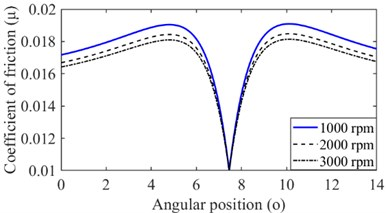The mesh stiffness of spur gear pair is simulated with Matlab, and the mixed EHL friction coefficient is applied in the model, the results are shown in Fig. 4, it can be drawn from the Fig. 4(a) that the meshing process of spur gear pair experiences single teeth meshing and double teeth meshing, as the mesh stiffness also fluctuates with the number of teeth in meshing. Furthermore, the amplified figure of the mesh stiffness in single teeth meshing shows that the mesh stiffness experiences abrupt change caused by the change of direction of friction force.

Fig. 4The mesh stiffness under EHL friction: a) original, b) amplified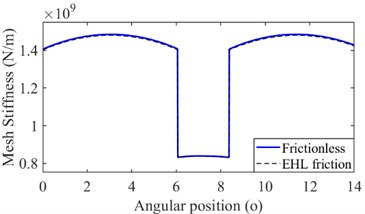a)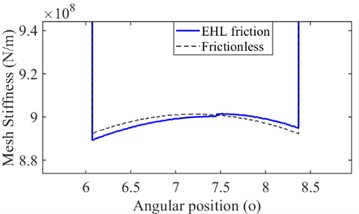b)

The mesh stiffness with spalling effect are also studied as shown in Fig. 5, in the section, the effect of height of spalled area is studied, the mesh stiffness of spur gear pair with spalled area heighted 3 mm, 5 mm, 8 mm, 10 mm, while the length and width of spalled area are all kept as 5mm, in addition, the spalled area happed nearby the pitch line. As the figure illustrate, the extent of drop for mesh stiffness in single teeth meshing area deepened as the height of spalled area expanded.

Fig. 5The mesh stiffness with spalling effect: a) hs = 3 mm, b) hs = 5 mm, c) hs = 8 mm, d) hs = 10 mm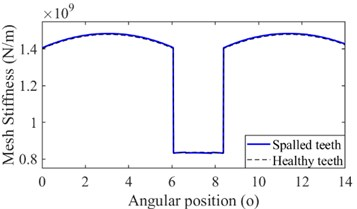a)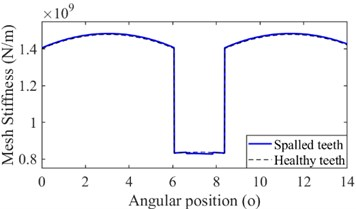b)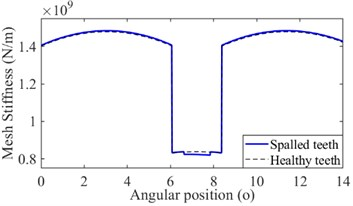c)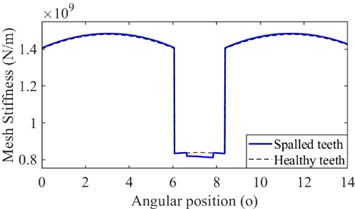d)

## 4. Conclusions

In this paper, the improved mesh stiffness calculation model that considered friction force excitation that obtained from the mixed EHL model, in addition, the spalling effect of the spur gear pair are also studied.

Form the results of numerical simulation, the mesh stiffness, especially in single teeth meshing, shows similar pattern as the evolution of friction coefficient. The sudden drop of mesh stiffness is illustrated as the spalling effect is considered, and the results show that the extent of dropping in mesh stiffness is amplified with the increase of height of the spalled area.

#### Acknowledgements

The authors are grateful for the financial support provided by the National Key R&D Program of China (Grant No. 2018YFB2001300); the National Natural Science Foundation of China (Grant No. 51775265, Grant No. 51775277). In addition, the authors declare that there is no conflict of interests regarding the publication of this article.